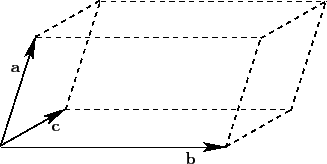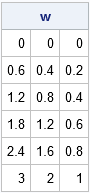A grid is a set of evenly spaced points. You can use SAS to create a grid of points on an interval, in a rectangular region in the plane, or even in higher-dimensional regions like the parallelepiped shown at the left, which is generated by three vectors. You can use vectors, matrices, and functions in the SAS/IML language to obtain a grid in a parallelepiped.

### Evenly spaced points in an interval

In one dimension, you can use a DO loop in the SAS DATA step to construct evenly spaced points in an interval. In the SAS/IML language, you can construct a vector of evenly spaced values operation without writing a DO loop. You can use the DO function, or you can use the colon operator (which SAS/IML documentation calls the "index creation operator"). The results are equivalent, as shown in the following program:

```proc iml; a = 2; b = 5; N = 5; delta = (b-a) / N; y = do(a, b, delta); /* Method 1: DO function: start=1, stop=b, step=delta */ x = a + delta*(0:N); /* Method 2: Use colon operator and vector sum */ print y, x;```### Subdivide a vector subspace

The second method generalizes to any linear subspace. For example, suppose you are given a vector u with magnitude α = ||u||. Then the following SAS/IML statements create points that are evenly spaced points along the vector u. The magnitudes of the N vectors are 0, α/N, 2α/N, and so forth up to α.

```/* linear spacing along a vector */ u = {3 2 1}; delta = u / N; /* Note: delta is vector */ w = delta @ T(0:N); /* i_th row is (i-1)*u */ print w;```It's always exciting to use the Kronecker product operator (@). The Kronecker product operator multiplies delta by 0, 1, 2, ..., N and stacks the results. Notice that the ith column of the w matrix is a linear progression from 0 to the ith component of u. Each row is a vector in the same direction as u.

### Grids in a linear subspace

It is only slightly harder to generate a grid of points in two or higher dimensions. In the DATA step, you can use nested DO loops to generate a grid of points in SAS. In the SAS/IML language, you can use the EXPANDGRID function.

Generate evenly spaced grid points in SAS. #SAStip
Click To Tweet

In fact, with the help of linear algebra, the EXPANDGRID function enables you to construct a grid in any linear subspace. Recall that a linear subspace is the "span," or set of all linear combinations, of k basis vectors.

From a linear algebra perspective, a rectangular grid is a set of discrete, evenly spaced, linear combinations of the standard Cartesian basis vectors e1, e2, ... ek. In a linear algebra course you learn that a matrix multiplication enables you to change to another set of basis vectors.

To give a concrete example, consider a rectangular grid of points in the square [0,1]x[0,1]. In the following SAS/IML program, the rows of the matrix G contain the grid points. You can think of each row as being a set of coefficients for a linear combination of the basis vectors e1 and e2. You can use those same coefficients to form linear combinations of any other basis vectors. The program shows how to form a grid in the two-dimensional linear subspace that is spanned by the vectors u = { 3 2 1} and v = {-3 5 2}:

```h = 1/N; /* generate linear combinations of basis vectors e1 and e2 */ G = ExpandGrid( do(0,1,h), do(0,1,h) );   u = { 3 2 1}; v = {-3 5 2}; M = u // v; /* create matrix of new basis vectors */ grid = G * M; /* grid = linear combinations of basis vectors u and v */```In the graph, the three-dimensional vectors u and v are indicated. The grid of points is a set of linear combinations of the form (i/N) u + (j/N) v, where 0 ≤ i, j ≤ N. The grid lies on a two-dimensional subspace spanned by u and v. The points in the grid are colored according to the values of the third component: blue points (lower left corner) correspond to low z-values whereas red points (upper right corner) correspond to high z-values.

Grids are important for visualizing multivariate functions. The function in this example is linear, but grids also enable you to visualize and understand nonlinear transformations.

The post Grids and linear subspaces appeared first on The DO Loop.